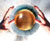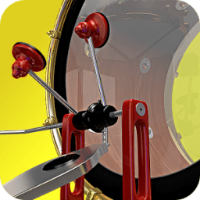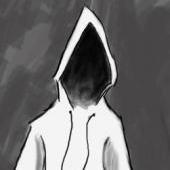1.## old school

Members

4

• ### Posts

1,063

2.## Atom

Members

1

• ### Posts

3,730

3.## jon3de

Members

1

• ### Posts

185

4.Members

1

300

## Popular Content

Showing content with the highest reputation on 03/11/2020 in all areas

1. Is there a way to art direct the "voronoifracturepoints"? How can I control where it places the groups of points?
1 point
2. Two more renders :
1 point
3. It's working and it's easy. What do you dislike, using uvs or using noises ? or another reason ? Volume_Pattern_01 F.hip
1 point
4. I sometimes have extremely slow nodes and deleting the contents of 'houdini_temp' in User/<Username>/AppData/Local/temp has always resolved it.
1 point
5. More fun 13.lesson. projectione.h /*! \fn vector2 one_sphere(vector2 z; float r) \brief Project \f$z \in C\f$ to \f$S^1\f$. Project \f$z \in C\f$ to \f$S^1\f$ with radius \f$r\f$. \param z the direction in \f$C\f$ to project to \f$S^1\f$ \param r the radius of \f$S^1\f$ \return the point \f$z \frac{r}{\mid z \mid}\f$ */ vector2 one_sphere(vector2 z; float r) { return z / length(z) * r; } /*! \fn vector two_sphere(vector z; float r) \brief Project \f$z \in R^3\f$ to \f$S^2\f$. Project \f$z \in R^3\f$ to \f$S^2\f$ with radius \f$r\f$. \param z the direction in \f$R^3\f$ to project to \f$S^2\f$ \param r the radius of \f$S^2\f$ \return the point \f$z \frac{r}{\mid z \mid}\f$ */ vector two_sphere(vector z; float r) { return z / length(z) * r; } /*! \fn vector2 stereo2(vector c) \brief Stereographic projection from \f$S^2\f$. Stereographic projection from \f$S^2\f$ into \f$C\f$. \param c the vector in \f$S^2\f$ to project into \f$C\f$, , must not be \f$(0, 0, 1)\top\f$ \return the stereographic projection to \f$C\f$ */ vector2 stereo2(vector c) { float x = c.x; float y = c.y; float z = c.z; return set(x, y) / (1-z); } /*! \fn vector stereo3(vector4 c) \brief Stereographic projection from \f$S^3\f$. Stereographic projection from \f$S^3\f$ into \f$R^3\f$. \param c the vector in \f$S^3\f$ to project into \f$R^3\f$, must not be \f$(0, 0, 0, 1)\top\f$ \return the stereographic projection to \f$R^3\f$ */ vector stereo3(vector4 c) { float x = c.x; float y = c.y; float z = c.z; float w = c.w; return set(x, y, z) / (1-w); } /*! \fn vector4 stereo3_inv(vector c) \brief Inverse of the stereographic projection from \f$S^3\f$. Inverse from \f$R^3\f$ into \f$S^3\f$ of the stereographic projection. \param c the vector in \f$R^3\f$ to reproject into \f$S^3\f$. \return the projection into \f$S^3\f$ */ vector4 stereo3_inv(vector c) { float x = c.x; float y = c.y; float z = c.z; return set(2*x, 2*y, 2*z, length2(c)-1) / (length2(c)+1); } vector stereo3_e4(vector4 c) { return set(c.x, c.y, c.z) / (1.-c.w); } vector stereo3_e4_inv(vector c) { return set(2*c.x, 2*c.y, 2*c.z, length2(c)-1) / (length2(c)+1.); } /*! \fn vector sphere_inversion(vector z; vector center; float scale) \brief Sphere inversion Perform a Möbius tranformation to project every point inside the the unit sphere in \f$R^3\f$ to outside and vice versa. \param z the original vector in \f$R^3\f$ \param center the center of the sphere \param scale the radius of the sphere \return the inverted vector */ vector sphere_inversion(vector z; vector center; float scale) { // Compute translation, then transform in the origin and retranslate vector translation = set(center.x, center.y, center.z); vector transformed = z - translation; return transformed / length2(transformed) * pow(scale, 2) + translation; } complex.h *! \fn vector2 cmul(vector2 z; vector2 w) \brief Multiply two complex numbers Multiply two arbitrary complex numbers. \param z first factor \param w second factor \return \f$z \cdot w\f$ */ vector2 cmul(vector2 z; vector2 w) { float x = z.x; float y = z.y; float u = w.u; float v = w.v; float real = x*u - y*v; float imaginary = x*v + y*u; return set(real, imaginary); } /*! \fn vector2 cdiv(vector2 w; vector2 z) \brief Divide one complex number by another complex number Divide one arbitrary complex number by another non-zero complex number. \param w divident \param z divison \return \f$\frac{w}{z}\f$ */ vector2 cdiv(vector2 w; vector2 z) { float x = z.x; float y = z.y; float u = w.u; float v = w.v; float divisor = pow(x, 2) + pow(y, 2); float real = (u*x + v*y) / divisor; float imaginary = (v*x - u*y) / divisor; return set(real, imaginary); } /*! \fn vector2 cpow(vector2 z; int n) \brief Compute the \f$n\f$-th power of a complex number Compute the \f$n\f$-th power of a complex number, for \f$n \in N\f$. \param z the complex number \param n the exponent \return \f$z^n\f$ */ vector2 cpow(vector2 z; int n) { float x = z.x; float y = z.y; float r = length(z); float phi = atan2(y, x); return pow(r, n) * set(cos(n*phi), sin(n*phi)); } /*! \fn float real(vector2 z) \brief Give the real part of a complex number Give the real part of a complex number represented by the first component of a two element vector. \param z the complex number \return the real part \f$a\f$ of \f$z = a + ib\f$ */ float real(vector2 z) { return z.x; } /*! \fn float img(vector2 z) \brief Give the imaginary part of a complex number Give the imaginary part of a complex number represented by the second component of a two element vector. \param z the complex number \return the imaginary part \f$b\f$ of \f$z = a + ib\f$ */ float img(vector2 z) { return z.y; } /*! \fn vector2 e_to_the_is(float s) \brief Give a point on the one-sphere. Give a point on the one-sphere parameterized by \f$s\f$ in the parametric form. \param s the real parameter \return the point \f$(\cos s, \sin s) \subset C\f$ */ vector2 e_to_the_is(float s) { return set(cos(s), sin(s)); } vector4 f; p@f; float n = chi("n"); float k = chi("k"); int j = @ptnum; float cosine = cos(($PI*j) / (2.*n)); float sine = sin(($PI*j) / (2.*n)); vector2 cosine_exp = cosine * e_to_the_is($PI -$PI/(k+1)); vector2 exp_neg = e_to_the_is(- $PI/4); vector2 exp_pos = e_to_the_is($PI/4); vector2 sine_exp_neg = sine * exp_neg; vector2 sine_exp_pos = sine * exp_pos; float j_mod_4n = j % (4*n); if( j_mod_4n < n ) { f.x = cosine; f.y = 0; f.z = real(sine_exp_neg); f.w = img(sine_exp_neg); } else { if( j_mod_4n < 2 * n ) { f.x = real(cosine_exp); f.y = img(cosine_exp); f.z = real(sine_exp_neg); f.w = img(sine_exp_neg); } else { if( j_mod_4n < 3 * n ) { f.x = real(cosine_exp); f.y = img(cosine_exp); f.z = real(sine_exp_pos); f.w = img(sine_exp_pos); } else { if( j_mod_4n < 4 * n ) { f.x = real(cosine); f.y = 0; f.z = real(sine_exp_pos); f.w = img(sine_exp_pos); } else { f.x = cosine; f.y = 0; f.z = real(sine_exp_pos); f.w = img(sine_exp_pos); } } } } p@f = f;
1 point
6. Thanks for the additional information. I can see the exterior band voxel bounding box change, when alter the value. I never really played around with that. Getting closer, though.
1 point
7. 1 point
8. sticking behavior is supported in new POPs, you just need to provide pospath, posprim, posuv and stuck attributes which is quite straightforward using scatter and attribcreate ts_pop_stick_release.hip
1 point
9.## get rid of mushroom effect in explosion and add details in the opening

1 point
10.## get rid of mushroom effect in explosion and add details in the opening

1 point
11.## get rid of mushroom effect in explosion and add details in the opening

1 point
12.